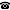# 2017 seminar talk: Bounded arithmetic and restricted reduced products

Talk held by Michal Garlík (Czech Academy of Sciences) at the KGRC seminar on 2017-01-26.

### Abstract

We present a construction of models of bounded arithmetic that yields nonelementary extensions but does not introduce new lengths. The construction has the form of a restricted reduced product. As an application we show that under the assumption of the existence of a one-way permutation $g$ hard against polynomial-size circuits, two similar-looking bounded arithmetic theories, $strict R^1_2(g)$ and $R^1_2(g)$, are in fact distinct. In particular, if such a permutation is definable by a term in the language of $R^1_2$, then $strict R^1_2$ is weaker than $R^1_2$. We discuss some strengthenings of this result.

Kurt Gödel Research Center for Mathematical Logic. Währinger Straße 25, 1090 Wien, Austria.+43-1-4277-50501. Last updated: 2010-12-16, 04:37.